# Common ion effect

Ionic Equilibrium: Common ion effect

Common ion effect occurs when a weak acid and its conjugate base or a weak base/conjugate acid are both present in a solution. It is a special case of the LeChatelier principle. When a common ion is added to a weak acid/base, is equivalent to adding stress to the system. Common ion effect is the suppression of ionization of a weak electrolyte by the presence in the same solution of a strong electrolyte containing one of the same ions as the weak electrolyte. The system responds to the stress induced by decreasing the solubility of one of the ions while keeping the Ksp constant. Likewise, if a solution and a salt to be dissolved in it have an ion in common, then the solubility of the salt is depressed relative to pure.

Consider the following general equation for precipitation of salt in its solution.

AB (s) ↔A+ (aq) + B (aq)

In this case, if you add BH(aq), which dissociates into B and H+, then the [A+]decreases, and AB is driven out of solution.

AgCl(s) ↔Ag+(aq) + Cl(aq)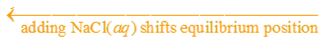Buffering is one of the most important applications of acid-base solutions containing a common ion.A buffered solution is one that resists a change in its pH when either hydroxide or protons are added.They essentially resist any pH changes, even when a small amount of strong acid or base is added to the buffer.Figure 1: Calculation involved to analyses the reaction when a strong acid or base is added to a buffer.

As shown in the figure above, suppose a buffered solution contains relatively large amounts of a weak acid HX and its conjugate base X.When a strong base (OH) is added to the solution since the weak acid is the best source of protons (H+), the following reaction occurs:

OH + HA → A + H2O

The net result is that OH ions are not allowed to accumulate but are replaced by A ions.

Similarly, if protons (H+) are added to a buffered solution of a weak acid and a salt of its conjugate base, the added protons react with A to form a weak acid, HA and free H+ ions do not accumulate.

H+ + A → HA

If [A] and [HA] are large with respect to the [H+] added, a small change in the pH is observed. In conclusion, if Ka is small (weak acid) then [H+] does not change much when [HX] and [X] change. If [HX] and [A] are large, and [HX]/[X] ≈ 1,then small additions of acid ([H+]) or base ([OH]) don’t change the ratio much. When the OH is added to the solution, the concentrations of HA and A change by small amounts. Under these conditions the [HA]/[A] ratio and thus the [H+] stay virtually constant(pH = pKa ± 1).

Example: Consider a 1.0 L of 0.10 M solution of CH3COOH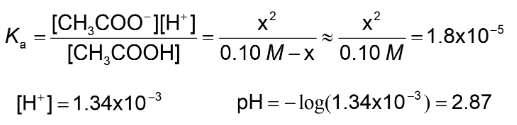When 0.01 mol of CH3COONa is added to1.0 L of 0.10 M solution of CH3COOH to make a buffer solution.

Recall the Henderson-Hasselbalch equation: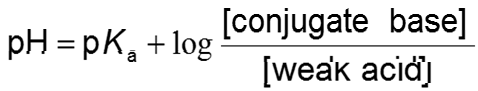When 0.001 mol of HCl, a strong acid is added to this buffer system: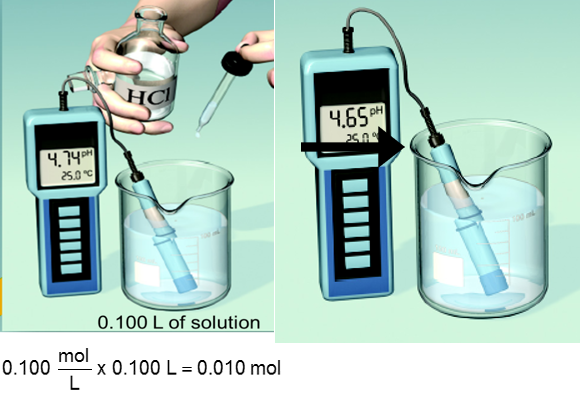When 0.001 mol of NaOH, a strong base is added to the system: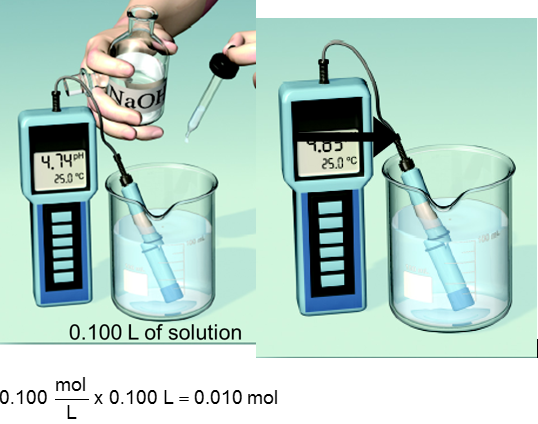.